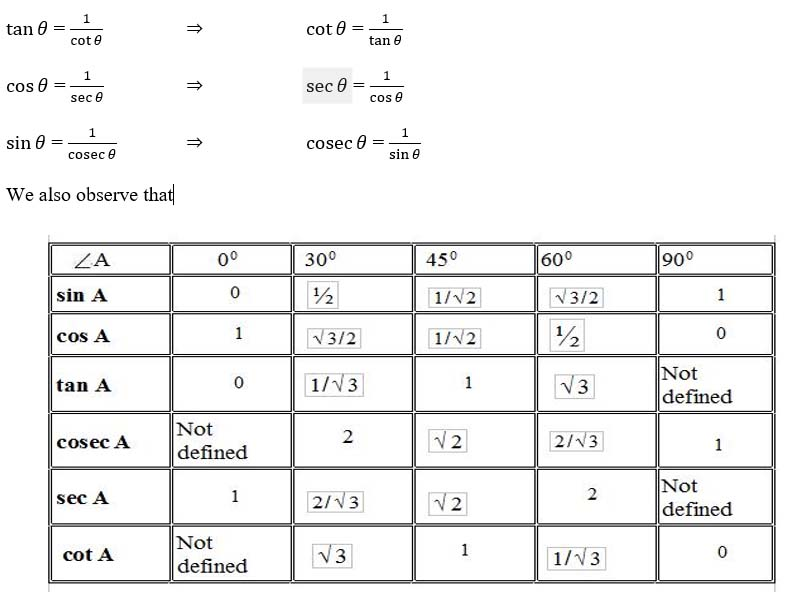# CBSE Worksheet for chapter- 9 Trigonometry class 10

## Find CBSE Worksheet for chapter- 9 Trigonometry class 10

CLASS-10

BOARD: CBSE

Mathematic Worksheet - 9

TOPIC: Trigonometry

For other CBSE Worksheet for class 10 Mathematic check out main page of Physics Wallah.

SUMMARYtan θ= sin θ/cos⁡θ and cotθ =cos⁡θ /sin θ

Trigonometric table:

Trigonometric Identities:

1. sin2θ + cos2θ = 1
2. 1 + tan2θ = sec2θ
3. 1 + cot2θ = cosec2θ

### Trigonometric ratios of complementary angles:

• Sin (900- θ) = cos θ
• cos (900- θ) = sin θ
• Tan (900- θ) = cot θ
• Cot (900- θ) = tan θ
• Sec (900- θ) = cosec θ
• Cosec (900- θ) = sec θ

#### OBJECTIVE

1. If 5tan θ = 4, then value of is : (5 sin⁡θ-3 cosθ)/(5 sinθ+3 cosθ)

1. 1/3
2. 1/6
3. 4/5
4. 2/3

2. Given 3sin β + 5cos β = 5, then the value of (3cos β- 5sin β)2 is equal to:

1. 9
2. 9/5
3. 1/3
4. 1/9

3. If tan θ= 4, then (tan⁡θ/(sin3 θ)/cosθ+sinθcosθ))is equal to:

1. 0
2. 2√2
3. √2
4. 1

4. As x increases from 0 to π/2 , the value of cos x :

1. Increases
2. decreases
3. remains constant
4. increases, then decreases

5. Value of x from the equation

x sin⁡ π/6 cos2 π/4 = (cot2 π/6 sec π/3 tan π/4)/(cosec2 π/4 cosec π/6) is :

1. 4
2. 6
3. -2
4. 0

6. The area of a triangle is 12 sq. cm. Two sides are 6 cm and 12 cm. The included angle is :

1. cos-1(1/3)
2. cos-1(1/6)
3. cos-1(1/6)
4. Sin-1(1/3)

7. If α + β = 900 and α = 2β, then cos2 + sin2β equals to:

1. 1/2
2. 0
3. 1
4. 2

SUBJECTIVE

1. If A + B = 900, prove that:
√(tanAtanB+tanAcotB)/sinAsecB-(sin2 B)/(cos2 A)=tanA
2. tanθ/(1-cotθ) +cotθ/(1-tanθ) = secθcosecθ+1
3. (sinA+cosA)/(sinA-cosA)+ (sinA-cosA)/(sinA+cosA)= 2/(sin2 A-cos2 A)=2/(1-2cos2 A)
4. ( sin8θ-cos8θ) = (sin2θ-cos2θ)(1-sin2 θcos2 θ)
5. (tanθ+secθ-1)/(tanθ-secθ+1) =(1+sinθ)/cosθ
6. If x = r sin θ cos φ, y = r sin θ sin φ, z = r cos θ, then prove that: x2+y2+z2=r2.
7. Prove that:
1/(secx-tanx)-1/cosx=1/cosx-1/(secx+tanx)
8. Evaluate: tan 70 tan 230 tan 600 tan 670 tan 830 + cot⁡540 /tan 360 + sin 200 sec 700- 2
9. Evaluate: cosec2580 - cot 580tan 320 - tan 130 tan 370 tan 450 tan 530 tan 770.
10. If 5x = sec θ and = tanθ, find the value of 5 (x2-1/x2 ).
11. Find the value of sec 600 geometrically.
12. Prove the following: sin A(1 + tan A) + cos A(1 + cot A) = sec A + cosec A

Objective:

1. b
2. a
3. d
4. b
5. b
6. d
7. a

Subjective:

1. √3
2. -1
3. 2